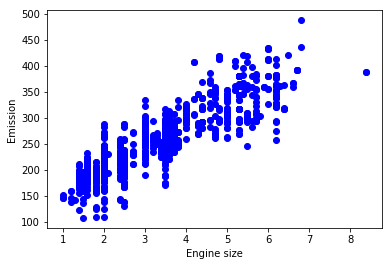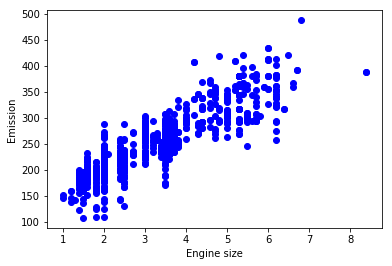# Multiple Linear Regression

#### About this Notebook

In this notebook, we learn how to use scikit-learn to implement Multiple linear regression. We download a dataset that is related to fuel consumption and Carbon dioxide emission of cars. Then, we split our data into training and test sets, create a model using training set, Evaluate your model using test set, and finally use model to predict unknown value

# Table of contents

### Importing Needed packages¶

In :
import matplotlib.pyplot as plt
import pandas as pd
import pylab as pl
import numpy as np
%matplotlib inline


### Downloading Data¶

To download the data, we will use !wget to download it from IBM Object Storage.

In :
!wget -O FuelConsumption.csv https://s3-api.us-geo.objectstorage.softlayer.net/cf-courses-data/CognitiveClass/ML0101ENv3/labs/FuelConsumptionCo2.csv

--2019-04-15 11:01:48--  https://s3-api.us-geo.objectstorage.softlayer.net/cf-courses-data/CognitiveClass/ML0101ENv3/labs/FuelConsumptionCo2.csv
Resolving s3-api.us-geo.objectstorage.softlayer.net (s3-api.us-geo.objectstorage.softlayer.net)... 67.228.254.193
Connecting to s3-api.us-geo.objectstorage.softlayer.net (s3-api.us-geo.objectstorage.softlayer.net)|67.228.254.193|:443... connected.
HTTP request sent, awaiting response... 200 OK
Length: 72629 (71K) [text/csv]
Saving to: ‘FuelConsumption.csv’

FuelConsumption.csv 100%[=====================>]  70.93K  --.-KB/s   in 0.04s

2019-04-15 11:01:48 (1.63 MB/s) - ‘FuelConsumption.csv’ saved [72629/72629]



Did you know? When it comes to Machine Learning, you will likely be working with large datasets. As a business, where can you host your data? IBM is offering a unique opportunity for businesses, with 10 Tb of IBM Cloud Object Storage: Sign up now for free

## Understanding the Data

### FuelConsumption.csv:¶

We have downloaded a fuel consumption dataset, FuelConsumption.csv, which contains model-specific fuel consumption ratings and estimated carbon dioxide emissions for new light-duty vehicles for retail sale in Canada. Dataset source

• MODELYEAR e.g. 2014
• MAKE e.g. Acura
• MODEL e.g. ILX
• VEHICLE CLASS e.g. SUV
• ENGINE SIZE e.g. 4.7
• CYLINDERS e.g 6
• TRANSMISSION e.g. A6
• FUELTYPE e.g. z
• FUEL CONSUMPTION in CITY(L/100 km) e.g. 9.9
• FUEL CONSUMPTION in HWY (L/100 km) e.g. 8.9
• FUEL CONSUMPTION COMB (L/100 km) e.g. 9.2
• CO2 EMISSIONS (g/km) e.g. 182 --> low --> 0

## Reading the data in

In :
df = pd.read_csv("FuelConsumption.csv")

# take a look at the dataset
df.head()

Out:
MODELYEAR MAKE MODEL VEHICLECLASS ENGINESIZE CYLINDERS TRANSMISSION FUELTYPE FUELCONSUMPTION_CITY FUELCONSUMPTION_HWY FUELCONSUMPTION_COMB FUELCONSUMPTION_COMB_MPG CO2EMISSIONS
0 2014 ACURA ILX COMPACT 2.0 4 AS5 Z 9.9 6.7 8.5 33 196
1 2014 ACURA ILX COMPACT 2.4 4 M6 Z 11.2 7.7 9.6 29 221
2 2014 ACURA ILX HYBRID COMPACT 1.5 4 AV7 Z 6.0 5.8 5.9 48 136
3 2014 ACURA MDX 4WD SUV - SMALL 3.5 6 AS6 Z 12.7 9.1 11.1 25 255
4 2014 ACURA RDX AWD SUV - SMALL 3.5 6 AS6 Z 12.1 8.7 10.6 27 244

Lets select some features that we want to use for regression.

In :
cdf = df[['ENGINESIZE','CYLINDERS','FUELCONSUMPTION_CITY','FUELCONSUMPTION_HWY','FUELCONSUMPTION_COMB','CO2EMISSIONS']]
cdf.head(9)

Out:
ENGINESIZE CYLINDERS FUELCONSUMPTION_CITY FUELCONSUMPTION_HWY FUELCONSUMPTION_COMB CO2EMISSIONS
0 2.0 4 9.9 6.7 8.5 196
1 2.4 4 11.2 7.7 9.6 221
2 1.5 4 6.0 5.8 5.9 136
3 3.5 6 12.7 9.1 11.1 255
4 3.5 6 12.1 8.7 10.6 244
5 3.5 6 11.9 7.7 10.0 230
6 3.5 6 11.8 8.1 10.1 232
7 3.7 6 12.8 9.0 11.1 255
8 3.7 6 13.4 9.5 11.6 267

Lets plot Emission values with respect to Engine size:

In :
plt.scatter(cdf.ENGINESIZE, cdf.CO2EMISSIONS,  color='blue')
plt.xlabel("Engine size")
plt.ylabel("Emission")
plt.show()#### Creating train and test dataset¶

Train/Test Split involves splitting the dataset into training and testing sets respectively, which are mutually exclusive. After which, you train with the training set and test with the testing set. This will provide a more accurate evaluation on out-of-sample accuracy because the testing dataset is not part of the dataset that have been used to train the data. It is more realistic for real world problems.

This means that we know the outcome of each data point in this dataset, making it great to test with! And since this data has not been used to train the model, the model has no knowledge of the outcome of these data points. So, in essence, it’s truly an out-of-sample testing.

In :
msk = np.random.rand(len(df)) < 0.8
train = cdf[msk]
test = cdf[~msk]


#### Train data distribution¶

In :
plt.scatter(train.ENGINESIZE, train.CO2EMISSIONS,  color='blue')
plt.xlabel("Engine size")
plt.ylabel("Emission")
plt.show()## Multiple Regression Model

In reality, there are multiple variables that predict the Co2emission. When more than one independent variable is present, the process is called multiple linear regression. For example, predicting co2emission using FUELCONSUMPTION_COMB, EngineSize and Cylinders of cars. The good thing here is that Multiple linear regression is the extension of simple linear regression model.

In :
from sklearn import linear_model
regr = linear_model.LinearRegression()
x = np.asanyarray(train[['ENGINESIZE','CYLINDERS','FUELCONSUMPTION_COMB']])
y = np.asanyarray(train[['CO2EMISSIONS']])
regr.fit (x, y)
# The coefficients
print ('Coefficients: ', regr.coef_)

Coefficients:  [[10.55398564  7.83589194  9.49083236]]


As mentioned before, Coefficient and Intercept , are the parameters of the fit line. Given that it is a multiple linear regression, with 3 parameters, and knowing that the parameters are the intercept and coefficients of hyperplane, sklearn can estimate them from our data. Scikit-learn uses plain Ordinary Least Squares method to solve this problem.

#### Ordinary Least Squares (OLS)¶

OLS is a method for estimating the unknown parameters in a linear regression model. OLS chooses the parameters of a linear function of a set of explanatory variables by minimizing the sum of the squares of the differences between the target dependent variable and those predicted by the linear function. In other words, it tries to minimizes the sum of squared errors (SSE) or mean squared error (MSE) between the target variable (y) and our predicted output ($\stackrel{^}{y}$$\hat{y}$) over all samples in the dataset.

OLS can find the best parameters using of the following methods:

- Solving the model parameters analytically using closed-form equations
- Using an optimization algorithm (Gradient Descent, Stochastic Gradient Descent, Newton’s Method, etc.)

## Prediction

In :
y_hat= regr.predict(test[['ENGINESIZE','CYLINDERS','FUELCONSUMPTION_COMB']])
x = np.asanyarray(test[['ENGINESIZE','CYLINDERS','FUELCONSUMPTION_COMB']])
y = np.asanyarray(test[['CO2EMISSIONS']])
print("Residual sum of squares: %.2f"
% np.mean((y_hat - y) ** 2))

# Explained variance score: 1 is perfect prediction
print('Variance score: %.2f' % regr.score(x, y))

Residual sum of squares: 715.98
Variance score: 0.84


explained variance regression score:
If $\stackrel{^}{y}$$\hat{y}$ is the estimated target output, y the corresponding (correct) target output, and Var is Variance, the square of the standard deviation, then the explained variance is estimated as follow:

$\mathtt{\text{explainedVariance}}\left(y,\stackrel{^}{y}\right)=1-\frac{Var\left\{y-\stackrel{^}{y}\right\}}{Var\left\{y\right\}}$$\texttt{explainedVariance}(y, \hat{y}) = 1 - \frac{Var\{ y - \hat{y}\}}{Var\{y\}}$
The best possible score is 1.0, lower values are worse.

## Practice

Try to use a multiple linear regression with the same dataset but this time use FUEL CONSUMPTION in CITY and FUEL CONSUMPTION in HWY instead of FUELCONSUMPTION_COMB. Does it result in better accuracy?

In :
# write your code here
regr = linear_model.LinearRegression()
x = np.asanyarray(train[['ENGINESIZE','CYLINDERS','FUELCONSUMPTION_CITY','FUELCONSUMPTION_HWY']])
y = np.asanyarray(train[['CO2EMISSIONS']])
regr.fit (x, y)
print ('Coefficients: ', regr.coef_)
y_= regr.predict(test[['ENGINESIZE','CYLINDERS','FUELCONSUMPTION_CITY','FUELCONSUMPTION_HWY']])
x = np.asanyarray(test[['ENGINESIZE','CYLINDERS','FUELCONSUMPTION_CITY','FUELCONSUMPTION_HWY']])
y = np.asanyarray(test[['CO2EMISSIONS']])
print("Residual sum of squares: %.2f"% np.mean((y_ - y) ** 2))
print('Variance score: %.2f' % regr.score(x, y))

Coefficients:  [[10.66448127  7.38345472  6.31291384  2.82232368]]
Residual sum of squares: 717.06
Variance score: 0.84


Double-click here for the solution.

## Want to learn more?

IBM SPSS Modeler is a comprehensive analytics platform that has many machine learning algorithms. It has been designed to bring predictive intelligence to decisions made by individuals, by groups, by systems – by your enterprise as a whole. A free trial is available through this course, available here: SPSS Modeler

Also, you can use Watson Studio to run these notebooks faster with bigger datasets. Watson Studio is IBM's leading cloud solution for data scientists, built by data scientists. With Jupyter notebooks, RStudio, Apache Spark and popular libraries pre-packaged in the cloud, Watson Studio enables data scientists to collaborate on their projects without having to install anything. Join the fast-growing community of Watson Studio users today with a free account at Watson Studio

### Thanks for completing this lesson!

#### Author: Saeed Aghabozorgi

Saeed Aghabozorgi, PhD is a Data Scientist in IBM with a track record of developing enterprise level applications that substantially increases clients’ ability to turn data into actionable knowledge. He is a researcher in data mining field and expert in developing advanced analytic methods like machine learning and statistical modelling on large datasets.

Copyright © 2018 Cognitive Class. This notebook and its source code are released under the terms of the MIT License.